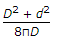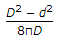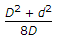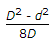# Civil Engineering - UPSC Civil Service Exam Questions - Discussion

Discussion Forum : UPSC Civil Service Exam Questions - Section 1 (Q.No. 7)
7.
A short column of external diameter D and internal diameter d, is subjected to a load W, with an eccentricity 'e', causing zero stress at an extreme fibre. Then the value of 'e' must beExplanation:
No answer description is available. Let's discuss.
Discussion:
12 comments Page 1 of 2.

Vikas said:   4 years ago
Thanks, @Ashwani.
(1)

Saqib khattak said:   4 years ago
As we know the formula for Z for ext and int dia is Z= 3.14(D^4-d^4)/32D and we also know that (D^4-d^4)= (D^2-d^2)(D^2+d^2) and we also know the area A= (3.14(D^2-d^2))/4
e=Z/A.

e=(3.14(D^4-d^4)/32D)/(3.14(D^2-d^2))/4.

Hence e will comes after solving.
e= (D^2+d^2)/8D.

Yempee said:   4 years ago
Thanks @Ashwani Bhandari.

PRAMOD KUMAR said:   5 years ago
@AFRID:

Z=I/y I= (π*D^4)/64 y=D/2 so Z = (π*D^3)/32 .

But as D-d cannot cancel out by D so the term remains same and only 64 cancelled out by 2 hence it is 32.

Ashwani bhandari said:   5 years ago
We know that e = (Z/A).
Section modulus for circular column Z = πx(D4-d4)÷(32D),
Area A= πx(D2-d2)÷4.

We know the formula of;
(D4-d4) is (D2+d2)( D2-d2),
Then e = Z ÷ A,
We get;
e = (D2+d2)/(8D).

Afrid said:   5 years ago
How come (32*D)?

Kavya said:   6 years ago
But here Z = (3.14*(D^4-d^4))/16D?

Chhaya said:   6 years ago
Z= (3.14* (D^4 - d^4))/32 D.
A = (3.14 * (D^2 - d^2))/4.
e= (D^2 + d^2)/8 D.

Asaithambi said:   7 years ago
σ(min) = 0 = (p/A) - (p.e/z),
e = z/A,
z = (3.14 * (D^2 - d^2))/(32 * D),
A = (3.14 * (D^2 - d^2),
σ(min)=(D^2 + d^2)/8D.

Vikas Niswade said:   7 years ago
Please provide the explanation if anyone can.